Function Repository Resource:

# PermutationFromIndex

Give the permutation corresponding to a given length and lexicographic index

Contributed by: Ed Pegg Jr
 ResourceFunction["PermutationFromIndex"][index,length] gives the permutation with a given lexicographic index and length.

## Details and Options

Permutations are considered to be in normal sorted order.
Lehmer codes are generated as an intermediary step.
The generating algorithm for Lehmer codes is as follows: For each number in the permutation, count how many subsequent values it exceeds.

## Examples

### Basic Examples (3)

Give the first permutation of length 4:

 In:=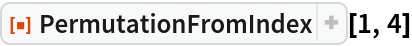Out=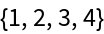Give the last permutation of length 4:

 In:=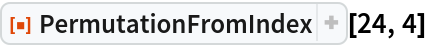Out=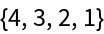Give the 777th permutation of length 7:

 In:=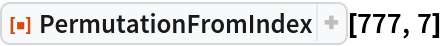Out=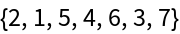### Scope (1)

Permutations with an arbitrarily large lexicographic index can returned:

 In:=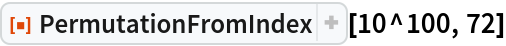Out=### Possible Issues (1)

When an index for length n exceeds n!, the index is considered mod n!:

 In:=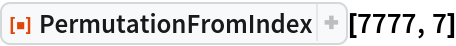Out=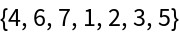In:=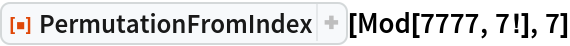Out=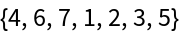### Neat Examples (1)

Permutations halfway through the index:

 In:=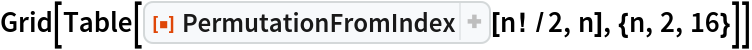Out=## Version History

• 1.0.0 – 20 December 2019